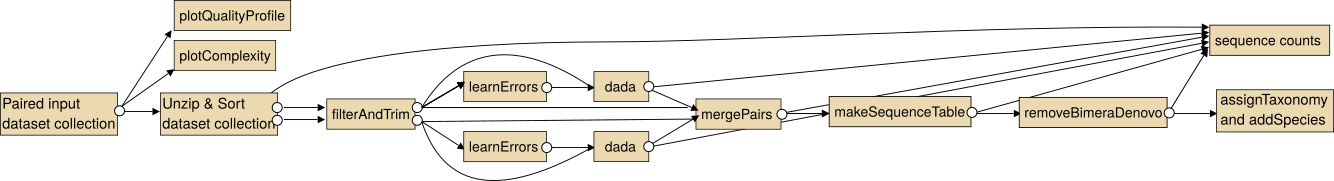Galaxy | Tool Preview

forward or reverse reads should be processed separately
Plotting Options
Plotting Option 0

# Description

Error rates are learned by alternating between sample inference and error rate estimation until convergence. Additionally a plot is generated that shows the observed frequency of each transition (eg. A->C) as a function of the associated quality score, the final estimated error rates (if they exist), the initial input rates, and the expected error rates under the nominal definition of quality scores.

In addition a plot is generated (with plotErrors) that shows the observed frequency of each transition (eg. A->C) as a function of the associated quality score. Also the final estimated error rates (if they exist) are shown. Optionally also the initial input rates and the expected error rates under the nominal definition of quality scores can be added to the plot.

# Usage

Input are the FASTQ dataset containing the filtered and trimmed reads of the samples.

Output a dataset with type dada2_errorrates (which is a RData file containing the output of dada2's learnErrors function) and a plot showing the error rates for each possible transition (A→C, A→G,...)

• Points are the observed error rates for each consensus quality score.
• The black line shows the estimated error rates after convergence of the machine-learning algorithm.
• The red line shows the error rates expected under the nominal definition of the Q-score.

# Details

The learnErrors method learns a parametric error model from the data, by alternating estimation of the error rates and inference of sample composition until they converge on a jointly consistent solution. As in many machine-learning problems, the algorithm must begin with an initial guess, for which the maximum possible error rates in this data are used (the error rates if only the most abundant sequence is correct and all the rest are errors).

It is expected that the estimated error rates (black lines in the plot) are in a good fit to the observed rates (points in the plot), and that the error rates drop with increased quality. Try to increase the number of bases to use for learning if this is not the case.

Error functions:

• loessErrfun: accepts a matrix of observed transitions, with each transition corresponding to a row (eg. row 2 = A->C) and each column to a quality score (eg. col 31 = Q30). It returns a matrix of estimated error rates of the same shape. Error rates are estimates by a loess fit of the observed rates of each transition as a function of the quality score. Self-transitions (i.e. A->A) are taken to be the left-over probability.
• noqualErrfun: accepts a matrix of observed transitions, groups together all observed transitions regardless of quality scores, and estimates the error rate for that transition as the observed fraction of those transitions. The effect is that quality scores will be effectively ignored.
• PacBioErrfun: This function accepts a matrix of observed transitions from PacBio CCS amplicon sequencing data, with each transition corresponding to a row (eg. row 2 = A->C) and each column to a quality score (eg. col 31 = Q30). It returns a matrix of estimated error rates of the same shape. Error rates are estimates by loessErrfun for quality scores 0-92, and individually by the maximum likelihood estimate for the maximum quality score of 93.

# Overview

The intended use of the dada2 tools for paired sequencing data is shown in the following image.Note: In particular for the analysis of paired collections the collections should be sorted lexicographical before the analysis.

For single end data you the steps "Unzip collection" and "mergePairs" are not necessary.